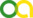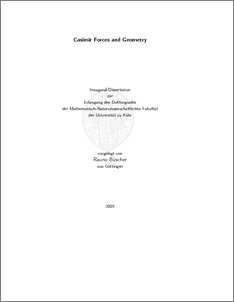Büscher, Rauno (2005). Casimir Forces and Geometry. PhD thesis, Universität zu Köln.Preview
PDF
dissertation_color.pdf

## Abstract

Casimir interactions are interactions induced by quantum vacuum fluctuations and thermal fluctuations of the electromagnetic field. Using a path integral quantization for the gauge field, an effective Gaussian action will be derived which is the starting point to compute Casimir forces between macroscopic objects analytically and numerically. No assumptions about the independence of the material and shape dependent contributions to the interaction are made. We study the limit of flat surfaces in further detail and obtain a concise derivation of Lifshitz' theory of molecular forces. For the case of ideally conducting boundaries, the Gaussian action will be calculated explicitly. Both limiting cases are also discussed within the framework of a scalar field quantization approach, which is applicable for translationally invariant geometries. We develop a non-perturbative approach to calculate the Casimir interaction from the Gaussian action for periodically deformed and ideally conducting objects numerically. The obtained results reveal two different scaling regimes for the Casimir force as a function of the distance between the objects, their deformation wavelength and -amplitude. The results confirm that the interaction is non-additive, especially in the presence of strong geometric deformations. Furthermore, the numerical approach is extended to calculate lateral Casimir forces. The results are consistent with the results of the proximity-force approximation for large deformation wavelengths. A qualitatively different behaviour between the normal and lateral force is revealed. We also establish a relation between the boundary induced change of the of the density of states for the scalar Helmholtz equation and the Casimir interaction using the path integral method. For statically deformed boundaries, this relation can be expressed as a novel trace formula, which is formally similar to the so-called Krein-Friedel-Lloyd formula. While the latter formula describes the density of states in terms of the S-matrix of quantum scattering at potentials, the new trace formula is applied to the free Green function, evaluated at the boundary surfaces of the confining geometry. This latter formulation is non-approximative and hence exact.

Item Type: Thesis (PhD thesis)
Creators:
CreatorsEmailORCIDORCID Put Code
Büscher, Raunorb@thp.uni-koeln.deUNSPECIFIEDUNSPECIFIED
URN: urn:nbn:de:hbz:38-15418
Date: 2005
Language: English
Faculty: Faculty of Mathematics and Natural Sciences
Divisions: Faculty of Mathematics and Natural Sciences > Department of Physics > Institute for Theoretical Physics
Subjects: Physics
Uncontrolled Keywords:
KeywordsLanguage
Casimir CasimireffektGerman
CasimirEnglish
Date of oral exam: 5 July 2005
Referee:View Item